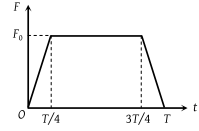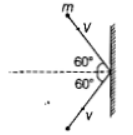Consider elastic collision of a particle of mass m moving with a velocity u with another particle of the same mass at rest. After the collision the projectile and the struck particle move in directions making angles θ1 and θ2 respectively with the initial direction of motion. The sum of the angles. θ1 + θ2, is

(1) 45°

(2) 90°

(3) 135°

(4) 180°

Concept Questions :-

Linear momentum
High Yielding Test Series + Question Bank - NEET 2020

Difficulty Level:

A particle of mass m moving with a velocity u makes an elastic one dimensional collision with a stationary particle of mass m establishing a contact with it for extremely small time T. Their force of contact increases from zero to F0 linearly in time $\frac{T}{4}$, remains constant for a further time $\frac{T}{2}$ and decreases linearly from F0 to zero in further time $\frac{T}{4}$ as shown. The magnitude possessed by F0 is(1) $\frac{mu}{T}$

(2) $\frac{2mu}{T}$

(2) $\frac{4mu}{3T}$

(4) $\frac{3mu}{4T}$

Concept Questions :-

Linear momentum
High Yielding Test Series + Question Bank - NEET 2020

Difficulty Level:

A rope is wound around a hollow cylinder of mass 3 kg and radius 40cm. What is the angular acceleration of the cylinder,if the rope is pulled with a force of 30 N?

(a) 25$m/{s}^{2}$

(b) 0.25 $rad/{s}^{2}$

(c) 25 $rad/{s}^{2}$

(d) 5 $m/{s}^{2}$

Concept Questions :-

Torque
High Yielding Test Series + Question Bank - NEET 2020

Difficulty Level:

Two discs of same moment of inertia rotating about their regular axis passing through centre and perpendicular to the plane of disc with angular velocities . They are brought into contact face to face coinciding the axis of rotation.  The expression for loss of energy during this proces is

(a)$\frac{1}{2}I{\left({\omega }_{1}+{\omega }_{2}\right)}^{2}$

(b) $\frac{1}{4}I{\left({\omega }_{1}-{\omega }_{2}\right)}^{2}$

(c) $I{\left({\omega }_{1}-{\omega }_{2}\right)}^{2}$

(d) $\frac{1}{8}I{\left({\omega }_{1}-{\omega }_{2}\right)}^{2}$

Concept Questions :-

Angular momentum
High Yielding Test Series + Question Bank - NEET 2020

Difficulty Level:

A rigid ball of mass m strikes a rigid wall at ${60}^{°}$ and gets reflected without loss of speed as shown in the figure. The value of impulse imparted by the wall on the ball will be(a) mv

(b) 2mv

(c) mv/2

(d) mv/3

Concept Questions :-

Linear momentum
High Yielding Test Series + Question Bank - NEET 2020

Difficulty Level:

A bullet of mass 10g moving horizontal with a velocity of 400 m/s strikes a wood block of mass 2 kg which is suspended by light inextensible string of length 5 m. As result, the centre of gravity of the block found to rise a vertical distance of 10 cm. The speed of the bullet after it emerges of horizontally from the block wiil be

(a) 100 m/s

(b) 80 m/s

(c) 120 m/s

(d) 160 m/s

Concept Questions :-

Linear momentum
High Yielding Test Series + Question Bank - NEET 2020

Difficulty Level:

Two identical balls A and B having velocities of 0.5 m/s and -0.3 m/s respectively collide elastically in one dimension. The velocities of B and A after the collision respectively will be

(a) -0.5 m/s and 0.3 m/s

(b) 0.5 m/s and -0.3 m/s

(c) -0.3 m/s and 0.5 m/s

(d) 0.3 m/s and 0.5 m/s

Concept Questions :-

Linear momentum
High Yielding Test Series + Question Bank - NEET 2020

Difficulty Level:

Two rotating bodies A and B of masses m and 2m with moments of inertia ${I}_{A}$ and ${I}_{B}\left({I}_{B}>{I}_{A}\right)$ have equal kinetic energy of rotation. If and ${L}_{B}$ be their angular momenta respectively, then

(a) ${L}_{A}=\frac{{L}_{B}}{2}$

(b) ${L}_{A}=2{L}_{B}$

(c) ${L}_{B}>{L}_{A}$

(d) ${L}_{A}>{L}_{B}$

Concept Questions :-

Angular momentum
High Yielding Test Series + Question Bank - NEET 2020

Difficulty Level:

A solid sphere of mass m and radius R is rotating about its diameter. A soild cyclinder of the same mass and same radius is also rotating about its geometrical axis with an angular speed twice that of the sphere. The ratio of their kinetic energies of rotation  will be

(a) 2:3

(b) 1:5

(c) 1:4

(d) 3:1

Concept Questions :-

Moment of inertia
High Yielding Test Series + Question Bank - NEET 2020

Difficulty Level:

A light rod of length l has two masses  attached to its two ends. The moment of inertia of the system about an axis perpendicular to the rod and passing through the centre of mass is

(a) $\frac{{m}_{1}{m}_{2}}{{m}_{1}+{m}_{2}}{l}^{2}$

(b)$\frac{{m}_{1}+{m}_{2}}{\sqrt{{m}_{1}{m}_{2}{l}^{2}}}{l}^{2}$

(c)$\left({m}_{1}+{m}_{2}\right){l}^{2}$

(d) $\sqrt{{m}_{1}{m}_{2}{l}^{2}}$

Concept Questions :-

Moment of inertia